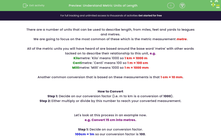# Understand Metric Units of Length

In this worksheet, students will convert between different units of length (such as millimetres, centimetres, metres, kilometres, etc.) to find equivalent distances which are identical but are expressed in different ways.Key stage:  KS 4

Year:  GCSE

GCSE Subjects:   Maths

GCSE Boards:   AQA, Eduqas, Pearson Edexcel, OCR,

Popular topics:   Geometry worksheets

Difficulty level:#### Worksheet Overview

There are a number of units that can be used to describe length, from miles, feet and yards to leagues and metres.

We are going to focus on the most common of these which is the metric measurement metre.

All of the metric units you will have heard of are based around the base word 'metre' with other words tacked on to describe their relationship to this unit, e.g.

Kilometre: 'Kilo' means 1000 so 1 km = 1000 m

Centimetre: 'Centi' means 100 so 1 m = 100 cm

Millimetre: 'Milli' means 1000 so 1 m = 1000 mm

Another common conversion that is based on these measurements is that 1 cm = 10 mm.

How to Convert

Step 1: Decide on our conversion factor (i.e. m to km is a conversion of 1000).

Step 2: Either multiply or divide by this number to reach your converted measurement.

Let's look at this process in an example now.

e.g. Convert 15 cm into metres.

Step 1: Decide on our conversion factor.

100cm = 1m so our conversion factor is 100.

Step 2: Multiply or divide by this number.

The way I look at this is that a metre is bigger than a cm, so there will be less of them overall.

As there is less of them, we need to divide.

15 ÷ 100 = 0.15 m

So 15 cm is the same as 0.15 m.

Let's practise converting in another example now.

e.g. Convert 1.4 km into metres.

Step 1: Decide on our conversion factor.

1000 m = 1 km so our conversion factor is 1000.

Step 2: Multiply or divide by this number.

The way I look at this one is that a metre is smaller than a km, so there will be more of them overall.

As there is more of them, we need to multiply.

1.4 x 1000 = 1400 m

So 1.4 km is the same as 1400 m.

In this activity, you will convert between different units of length (such as millimetres, centimetres, metres, kilometres, etc.) to find equivalent distances which are identical but are expressed in different ways.

### What is EdPlace?

We're your National Curriculum aligned online education content provider helping each child succeed in English, maths and science from year 1 to GCSE. With an EdPlace account you’ll be able to track and measure progress, helping each child achieve their best. We build confidence and attainment by personalising each child’s learning at a level that suits them.

Get started